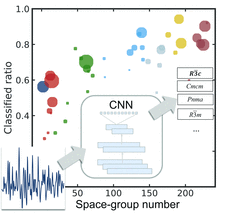# issue contentsFOUNDATIONSADVANCES
ISSN: 2053-2733

# July 2019 issueCover illustration: Whilst algorithms exist to determine the crystallographic space group of a sample from its single-crystal or powder diffraction pattern, none exist to accomplish this from a measured atomic pair distribution function (PDF). Logically speaking, the symmetry information is in the PDF, which is just a Fourier transform of the powder diffraction intensity, but the Fourier transform mixes the information up in non-intuitive ways. This problem is therefore ideal for machine learning, as demonstrated by Liu et al. [Acta Cryst. (2019), A75, 633-643]. A convolutional neural network (CNN) model was trained to take as input a PDF (lower left) and give as output a rank-ordered list of most probable space groups. The classification ratio is the number of correct classifications divided by the total number of data sets in the test set from that space group, and the size of the colored dots represents the number of PDFs in that space group in the training set.

## research papers

### Multipole electron densities and atomic displacement parameters in urea from accurate powder X-ray diffraction

The electron density of crystalline urea has been determined based on powder X-ray diffraction data.

## research papers

### Theoretical study of the properties of X-ray diffraction moiré fringes. II. Illustration of angularly integrated moiré images

Using a recently developed moiré-fringe theory of X-ray diffraction, the angularly integrated moiré images of a lightly strained silicon bicrystal having an interspacing gap were simulation-computed over a wide range of crystal thicknesses and incident-beam angular width.

### Temperature-dependent atomic B factor: an ab initio calculation

The calculation of the mean-square atomic displacements (B factors) implemented in the open source code thermo_pw is presented. The B factors can be computed within the harmonic or the quasi-harmonic approximation for an arbitrary material with the accuracy of ab initio methods. A simplified calculation based on the Debye model is also implemented, where the only input ingredient is the Debye temperature which can also be calculated ab initio by the software. Applications to silicon and to the hexagonal close-packed metals Mg, Ru and Cd are presented.

### Using a machine learning approach to determine the space group of a structure from the atomic pair distribution function

We present applications of machine learning models for predicting the space group of the underlying structure from its atomic pair distribution function (PDF).

### Complex structural ordering of the oxygen deficiency in La0.5Ca2.5Mn2O7−δ Ruddlesden–Popper phases

New oxygen-deficient manganese Ruddlesden–Popper-related phases, La0.5Ca2.5Mn2O6.5 and La0.5Ca2.5Mn2O6.25, have been synthesized by controlled reduction of the fully oxidized n = 2 term La0.5Ca2.5Mn2O7. A complete structural and compositional characterization allows the proposition of a topotactic reduction pathway through preferential oxygen removal in the [MnO2] layers along  directions.

### Theoretical study of the properties of X-ray diffraction moiré fringes. I. Corrigenda and addenda

Seven corrections are made and several supplementary equations are added to the article by Yoshimura [Acta Cryst. (2015), A71, 368–381].